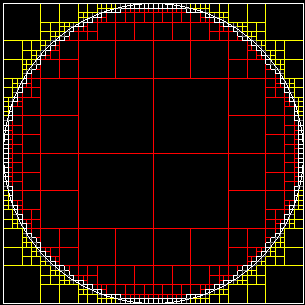﻿ Petro Protsyk personal page

# Approximation of circle with rectangles

Quad tree is an interesting data structure. It is used for solving various geometrical problems, such as fast collision detection, indexing of geospacial data, etc. I am going to show how it might be used for approximation of different 2D shapes. (There is also 3D version of quad trees, called octal tree).Small white rectangles approximate circle’s border. Red rectangles form an inner area of the circle. Yellow does not belong to the circle and might be removed.

Building quad tree is simple. We start from the bounding rectangle. Then it will be split into four smaller rectangles of the equal size. Let’s say, input rectangle is defined by following points (x1,y1) -> (x2,y2). Then rules for splitting it into four smaller rectangles are following:

• (x1, y1) -> (x2-width/2,y2-height/2)
• (x1, y1+height/2) -> (x1+width/2,y2)
• (x2-width/2, y2-height/2) -> (x2,y2)
• (x2-width/2, y1) -> (x2,y2-height/2)

This procedure might be recursively applied to all leafs in the tree until new leaf rectangles reach certain size.

In order to make a decision if given leaf rectangle should be split, we need a function f, which for a given rectangle r will return how many of its verteces belong to the figure. There are obviously four cases:

• f(r) = 4 – Rectangle belongs to the inner area of the figure. No further split required. (Such rectangles are red)
• f(r) = 3 or 2 – These rectangles touch the border of the figure and about half of the rectangle belongs to figure. (White rectangles)
• f(r) = 1 – Less than half of the rectangle belongs to figure. (Gray rectangles)
• f(r) = 0 – Rectangle lays outside the figure. No further split required. (Yellow rectangles)

For a circle, function f is quite simple:

• f(x1,y1,x2,y2) = belongs(x1,y1)+belongs(x1,y2)+belongs(x2,y2)+belongs(x2,y2),

Where

• belongs(x,y) = 1, if x*x+y*y< =r*r and 0 otherwise,
• r – is the radius of the circle.